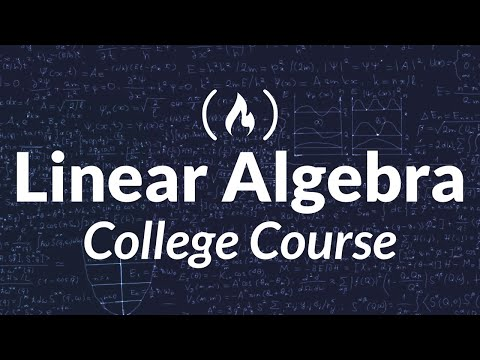Learn Linear Algebra in this 20-hour college course. Watch the second half here: https://youtu.be/DJ6YwBN7Ya8
This course is taught by Dr. Jim Hefferon, a professor of mathematics at St Michael’s College.

📔 The course follows along with Dr. Hefferon’s Linear Algebra text book. The book is available for free: http://joshua.smcvt.edu/linearalgebra/book.pdf

📚 Access additional course resources at: https://hefferon.net/linearalgebra/

🔗 Stephen Chew’s Learning How to Learn series: https://www.youtube.com/watch?v=htv6eap1-_M&list=PL85708E6EA236E3DB
🔗 3Blue1Brown’s Linear Algebra series: https://www.youtube.com/watch?v=fNk_zzaMoSs&list=PLZHQObOWTQDPD3MizzM2xVFitgF8hE_ab

⭐️ Course Contents ⭐️
⌨️ (0:00:00) Introduction to Linear Algebra by Hefferon
⌨️ (0:04:35) One.I.1 Solving Linear Systems, Part One
⌨️ (0:26:08) One.I.1 Solving Linear Systems, Part Two
⌨️ (0:40:56) One.I.2 Describing Solution Sets, Part One
⌨️ (0:54:21) One.I.2 Describing Solution Sets, Part Two
⌨️ (1:02:48) One.I.3 General = Particular + Homogeneous
⌨️ (1:18:33) One.II.1 Vectors in Space
⌨️ (1:35:08) One.II.2 Vector Length and Angle Measure
⌨️ (1:51:31) One.III.1 Gauss-Jordan Elimination
⌨️ (2:00:00) One.III.2 The Linear Combination Lemma
⌨️ (2:44:32) Two.I.1 Vector Spaces, Part One
⌨️ (3:08:12) Two.I.1 Vector Spaces, Part Two
⌨️ (3:33:01) Two.I.2 Subspaces, Part One
⌨️ (3:58:16) Two.I.2 Subspaces, Part Two
⌨️ (4:23:43) Two.II.1 Linear Independence, Part One
⌨️ (4:45:11) Two.II.1 Linear Independence, Part Two
⌨️ (5:03:57) Two.III.1 Basis, Part One
⌨️ (5:23:55) Two.III.1 Basis, Part Two
⌨️ (5:42:34) Two.III.2 Dimension
⌨️ (6:03:24) Two.III.3 Vector Spaces and Linear Systems
⌨️ (6:25:09) Three.I.1 Isomorphism, Part One
⌨️ (6:54:08) Three.I.1 Isomorphism, Part Two
⌨️ (7:21:47) Three.I.2 Dimension Characterizes Isomorphism
⌨️ (7:43:43) Three.II.1 Homomorphism, Part One
⌨️ (8:14:52) Three.II.1 Homomorphism, Part Two
⌨️ (8:30:24) Three.II.2 Range Space and Null Space, Part One
⌨️ (9:00:17) Three.II.2 Range Space and Null Space, Part Two.
⌨️ (9:20:57) Three.II Extra Transformations of the Plane
⌨️ (9:52:06) Three.III.1 Representing Linear Maps, Part One.
⌨️ (10:13:18) Three.III.1 Representing Linear Maps, Part Two
⌨️ (10:34:18) Three.III.2 Any Matrix Represents a Linear Map
⌨️ (10:58:32) Three.IV.1 Sums and Scalar Products of Matrices
⌨️ (11:19:14) Three.IV.2 Matrix Multiplication, Part One
⌨️ (11:39:44) Three.IV.2 Matrix Multiplication, Part Two

The following sections are in the second video: https://youtu.be/DJ6YwBN7Ya8
⌨️ Three.IV.3 Mechanics of Matrix Multiplication
⌨️ Three.IV.4 Matrix Inverse, Part One
⌨️ Three.IV.4 Matrix Inverse, Part Two
⌨️ Three.V.1 Changing Vector Representations
⌨️ Three.V.2 Changing Map Representations, Part One
⌨️ Three.V.2 Changing Map Representations, Part Two
⌨️ Three.VI Projection
⌨️ Four.I.1 Determinants
⌨️ Four.I.3 Permutation Expansion, Part One
⌨️ Four.I.3 Permutation Expansion, Part Two
⌨️ Four.I.4 Determinants Exist (optional)
⌨️ Four.II.1 Geometry of Determinants
⌨️ Four.III.1 Laplace’s formula for the determinant
⌨️ Five.I.1 Complex Vector Spaces
⌨️ Five.II.1 Similarity
⌨️ Five.II.2 Diagonalizability
⌨️ Five.II.3 Eigenvalues and Eigenvectors, Part One
⌨️ Five.II.3 Eigenvalues and Eigenvectors, Part Two
⌨️ Five.II.3 Geometry of Eigenvalues and Eigenvectors